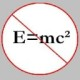You are currently browsing the tag archive for the ‘hard integral’ tag.

We got some very good response to our last week’s problem from several of our “regular” problem-solvers as well as several others who are “new”. There were solutions that were more “algebraic” than others, some that had a more “trigonometric” flavor to them and some that had a combination of both. All the solutions we received this time were correct and they all deserve to be published, but for the sake of brevity I will post just one.

Solution to POW-5: (due to Animesh Datta, Univ of New Mexico)

Note that the given integral may be written as$\displaystyle \int \frac{x^2 - 1}{x(x^2 + 1) \sqrt{x^2 + 1/x^2}} \, dx$$\displaystyle = \int \frac{1 - 1/x^2}{(x + 1/x) \sqrt{(x + 1/x)^2 - 2}} \, dx$.

Now, we use the substitution$t = x + 1/x$, which transforms the integral into$\displaystyle \int \frac1{t \sqrt{t^2 - 2}} \, dt$.

Finally, we use one last (trigonometric) substitution$t = \sqrt{2} \sec \theta$, which transforms the integral into$\displaystyle \int \frac1{\sqrt{2}} \, d\theta$, which evaluates to$\theta /\sqrt{2} + C$, which equals$\displaystyle \frac1{\sqrt2} \arctan \sqrt{\frac12 (x^2 + \frac1{x^2})} + C$. And this is our final answer!

Watch out for the next POW that will be posted by Todd!

Source: I had mentioned earlier that Carl Lira had brought this integral to our attention, and he in turn had found it in the MIT Integration Bee archives. This one was from the year 1994.

Trivia: Four out of the six people who sent correct solutions are either Indians or of Indian origin! Coincidence? 🙂

Time for our next problem in the POW series! Earlier, Todd and I deliberated for a bit on whether we should pose a “hard” Ramanujan identity (involving an integral and Gamma function) as the next POW, but decided against doing it. Perhaps, we may do so some time in the future.

Okay, the following integral was brought to our attention by Carl Lira, and for the time being I won’t reveal the actual source of the problem.

Compute$\displaystyle \int \frac{x^2 - 1}{(x^2 + 1) \sqrt{x^4 + 1}} \, dx$.

It is “hard” or “easy” depending on how you look at it!

Please send your solutions to topological[dot]musings[At]gmail[dot]com by Wednesday, June 26, 11:59pm (UTC); do not submit solutions in Comments. Everyone with a correct solution gets entered in our Hall of Fame! We look forward to your response.

### Blog Stats

• 357,711 hits

 Convictions ·… on Continued fraction for eJohn Favors on Solution to POW-13: Highly…Wayne J. Mann on Solution to POW-12: A graph co…erneststephen on The 54th Carnival of Math…anhtraisg on p^q + q^p is primeprof dr drd horia or… on My first postprof drd horia orasa… on My first postprof dr mircea orasa… on Inequality with lognotedscholar on Self-referential Paradoxes, In…prof dr mircea orasa… on Inequality with logprof dr mircea orasa… on Inequality with logprof dr mircea orasa… on 2010 in reviewkenji on Basic category theory, Iprof dr mircea orasa… on Solution to POW-10: Another ha…prof drd horia orasa… on Continued fraction for e